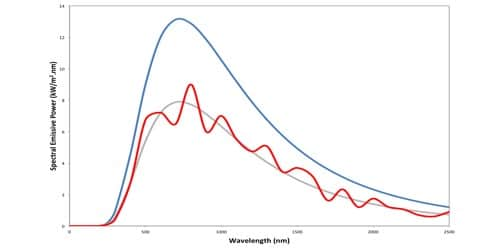Physics

# Define Emissive PowerThe emissive power of a body at a given temperature is the amount of energy emitted per unit time per unit area of the surface for a given wavelength. It is the energy of thermal radiation emitted in all directions per unit time from each unit area of a surface at any given temperature

The emissive power of a body is defined as the ratio of the amount of energy emitted (or radiated) per unit area per second to the amount of emitted heat energy per unit area of a perfectly black body per second at the same temperature. All bodies will emit heat when the body temperature is above absolute zero, and this is called emission and when this emitted heat travels in the form of electromagnetic wave it is called thermal radiation. This power is the energy of thermal radiation emitted in all directions per unit time per unit area of a surface at any given temperature.

If ‘Q’ is the amount of radiant energy emitted, ‘A’ is the surface area of the body and ‘t ‘ is the time for which body radiates energy, then the emissive power is –

E = Q/At

The coefficient of emission of a body is the ratio of the emissive power of the body at a given temperature to the emissive power of a perfectly black body at the same temperature. The value of emissive power lies between 0 to 1. The emissive power of a perfectly black body is 1. But, a perfectly white surface or body has the zero emissive power.

It is denoted by eλ.

Its unit is W m-2.

Coefficient of emission, e = E/Eb

The emissive power eλ of a body for radiation of wavelength λ to λ+dλ is the amount of radiation emitted per unit area of the body per second normally in-unit solid angle in the axial direction of the solid angle.